# Problem 1 (15%)¶

Consider provided Jupyter notebook Summaries and NameScopes.ipynb. Add one more output summary. For example, calculate the rolling mean of all one dimensional tensors passed as arguments of run_graph function. Provide working notebook and images of your graphs and calculated summaries. In the Word document presented as your solution provide snippets of additional or modified code.

### Moving average¶

I calculated the Cumulative Moving Average, i.e, the average of all vectors up to the current data. I also updated the output summary so that it includes the results from the Cumulative Moving Average.

In :
# Import library
import tensorflow as tf
import numpy as np
from IPython.display import Image

In :
# Helper function
def run_graph(input_tensor):
"""
Helper function; runs the graph with given input
tensor and saves summaries
"""
feed_dict = {a: input_tensor}
out, step, summary = sess.run([output,
increment_step, merged_summaries],
feed_dict=feed_dict)

In :
# Explicitly create a Graph object
graph = tf.Graph()

with graph.as_default():

with tf.name_scope("variables"):
global_step = tf.Variable(0, dtype=tf.int32, name="global_step")
total_length = tf.Variable(0, dtype=tf.int32, name="total_length")
total_sum = tf.Variable(0.0, dtype=tf.float32, name="total_sum")
total_output = tf.Variable(0.0, dtype=tf.float32, name="total_output")

# Primary transformation Operations
with tf.name_scope("transformation"):

# Separate input layer
with tf.name_scope("input"):
# Create input placeholder- takes in a Vector
a = tf.placeholder(tf.float32, shape=[None], name="input_placeholder_a")

# Separate middle layer
with tf.name_scope("intermediate_layer"):
b = tf.reduce_prod(a, name="product_b")
c = tf.reduce_sum(a, name="sum_c")
d = tf.size(a, name="length_d")

# Summary Operations
with tf.name_scope("summaries"):
roll_mean = tf.div(update_sum,
tf.cast(update_length, tf.float32),
name="Rolling_mean")
avg = tf.div(update_total, tf.cast(increment_step,
tf.float32),
name="average")

# Creates summaries for output node
tf.summary.scalar('1_Product', b)
tf.summary.scalar('2_Sum', c)
tf.summary.scalar('3_Rolling_mean', roll_mean)
tf.summary.scalar('4_Output', output)
tf.summary.scalar('5_Sum_of_outputs_over_time',
update_total)
tf.summary.scalar('6_Average_of_outputs_over_time',
avg)

# Global Variables and Operations
with tf.name_scope("global_ops"):
# Initialization Op
init = tf.global_variables_initializer()
# Merge all summaries into one Operation
merged_summaries = tf.summary.merge_all()

# Start a Session, using the explicitly created Graph
sess = tf.Session(graph=graph)

# Open a SummaryWriter to save summaries
writer = tf.summary.FileWriter('summaries', graph)

# Initialize Variables
sess.run(init)

In :
# Run the graph with various inputs
run_graph([2,8])
run_graph([3,1,3,3])
run_graph()
run_graph([1,2,3])
run_graph([11,4])
run_graph([4,1])
run_graph([7,3,1])
run_graph([6,3])
run_graph([0,2])
run_graph([4,5,6])

In :
# Write the summaries to disk
writer.flush()

# Close the tf.summary.FileWriter
writer.close()

# Close the session
sess.close()


### Plot I: Calculated summaries¶

In order to analyis the tensorboard graphics the following bash command can be run.

tensorboard --logdir 'summaries'


The following tensorgraphs show the 10 runs we're having with the vectors: [2,8], [3,1,3,3], , [1,2,3], [11,4], [4,1], [7,3,1], [6,3], [0,2], [4,5,6].

In :
fig = Image(filename=('/home/tim/hw11/img/p1_1.png'))
fig

Out: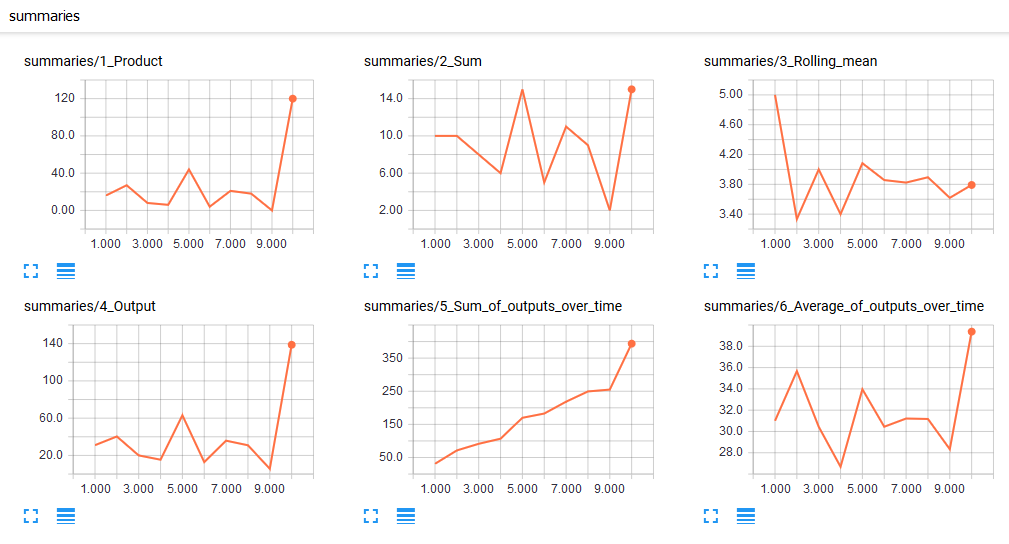#### Individual values (upper half)¶

The first picture shows the product of all the values inside the vector (e.g., vector nr. 1 has a value of 16). The second picture is the sum of the values inside the vector (e.g., vector nr. 1 has a value of 10). The third picture shows the rolling cumulative mean value. vector 1 has a mean value of 5, that means the cumulative rolling mean is also 5. Moving on to vector 2, the mean value is 2.5, however, the rolling mean is 3.33. This is because we're now having the combination of vector 1 and 2 to to consider, i.e., [2, 8, 3, 1, 3, 3]. The mean value of that is 3.33.

#### Aggregated values (lower part)¶

The lower part of the above pictures is showing the aggregated summary values. The fourth picture describes the overall sum of all values calculated inside the functions 1-3 (i.e., product + sum + rolling_mean). The fifth picture shows the overall sum over time (agg_ouput1 + agg_ouput1 etc.). The sixth pictures shows the mean of the outputs over the time.

### Plot II: Tensorgraph¶

The following picture shows the network graph of the analyis above.

In :
fig = Image(filename=('/home/tim/hw11/img/p1_2.png'))
fig

Out: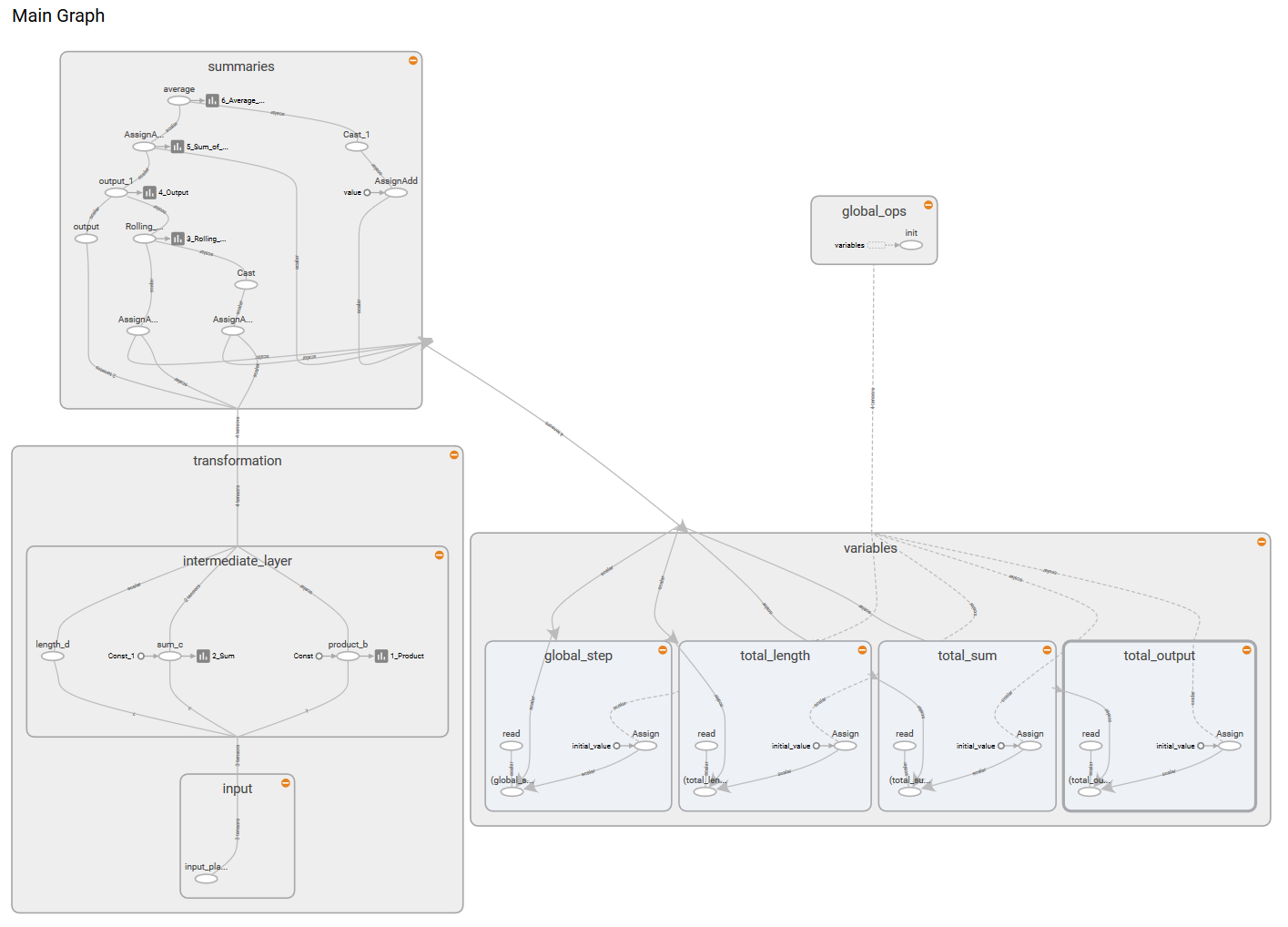# Problem 2 (25%)¶

Question: Consider the attached file logistic_regression_mnist.py. Search through TensorFlow API documentation and the Internet and describe for us what is the meaning and purpose of functions used in step 5 and step 6.

#### Meaning step 5¶

The tf.nn.softmax_cross_entropy_with_logits function in step 5 calculates the cross entropy of the result after using a softmax function. The loss function is defined as the mean of entropy.

#### Meaning step 6¶

In step 6 gradient decent is used in order to minimize the loss. In this step the model is trained on a certain batch size. Furthermore the average loss per batch is recorded with the total run time and the accuracy of the model using some test data.

Question: Demonstrate that you can run the code successfully.

We can run the logistic_regression_mnist-1.py code with the following bash command:

python /home/tim/hw11/code/logistic_regression_mnist-1.py


Having those parameters:

• learning_rate = 0.01
• batch_size = 128
• n_epochs = 30

2017-11-13 14:27:24.729610: I tensorflow/core/platform/cpu_feature_guard.cc:137] Your CPU supports instructions that this TensorFlow binary was not compiled to use: SSE4.1 SSE4.2 AVX AVX2 FMA
Average loss epoch 0: 1.28509407972
Average loss epoch 1: 0.732446798217
Average loss epoch 2: 0.599800021498
Average loss epoch 3: 0.537463494809
Average loss epoch 4: 0.497836460878
Average loss epoch 5: 0.471589082743
Average loss epoch 6: 0.451638019168
Average loss epoch 7: 0.435541740108
Average loss epoch 8: 0.423157613038
Average loss epoch 9: 0.412838064395
Average loss epoch 10: 0.405262912278
Average loss epoch 11: 0.397252654314
Average loss epoch 12: 0.390558742768
Average loss epoch 13: 0.383705951986
Average loss epoch 14: 0.378570248614
Average loss epoch 15: 0.374783192273
Average loss epoch 16: 0.371308067705
Average loss epoch 17: 0.366376711182
Average loss epoch 18: 0.362410888354
Average loss epoch 19: 0.358795878944
Average loss epoch 20: 0.358775554559
Average loss epoch 21: 0.35313237488
Average loss epoch 22: 0.350272573479
Average loss epoch 23: 0.348385391223
Average loss epoch 24: 0.347936009541
Average loss epoch 25: 0.343370734534
Average loss epoch 26: 0.343159333879
Average loss epoch 27: 0.339506200005
Average loss epoch 28: 0.33858445846
Average loss epoch 29: 0.33675496367
Total time: 19.3846440315 seconds
Optimization Finished!
Accuracy 0.9116


Question: Fetch for us the TensorBoard Graph.

In order to fetch the tensorboard graph we're running the following command:

tensorboard --logdir 'logistic_reg'

In :
fig = Image(filename=('/home/tim/hw11/img/p2_1.png'))
fig

Out: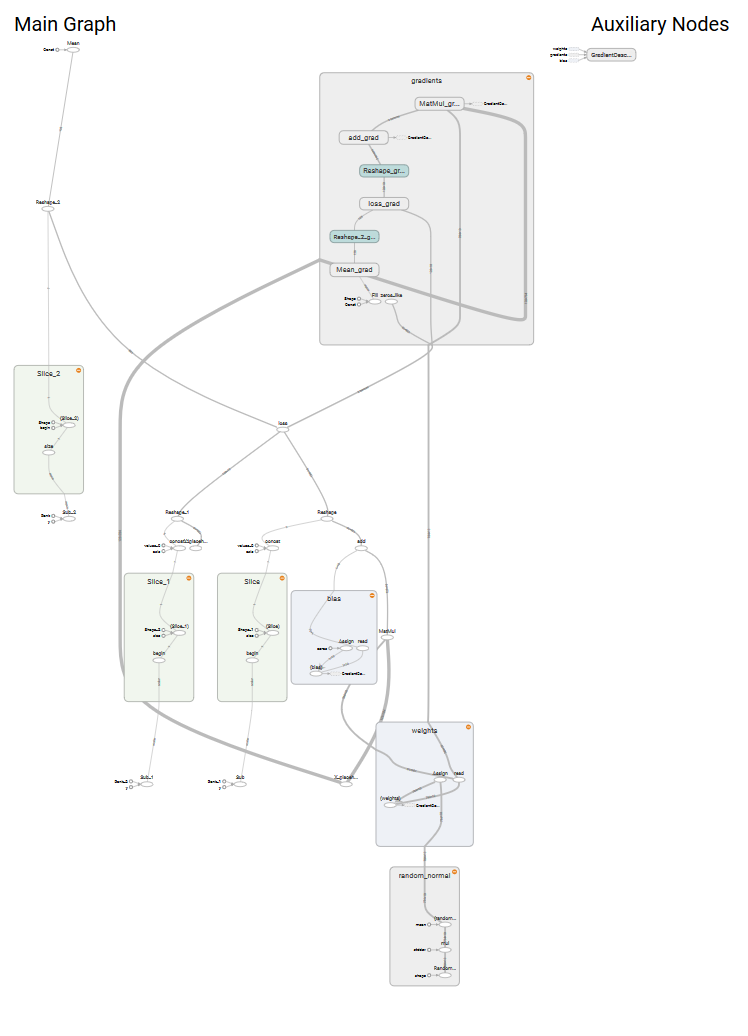Question: Vary parameter batch_size through values: 8, 64, 128, 256 and report and plot changes in the execution time and accuracy. Keep other parameters the same as in the original program.

In :
# Load libraries
import matplotlib.pyplot as plt
import tensorflow as tf
import numpy as np
from tensorflow.examples.tutorials.mnist import input_data
import time

In :
# Adapt training to allow for different variables
def run_training(learning_rate, batch_size, n_epochs, mnist):

# Step 2: create placeholders for features and labels
# each image in the MNIST data is of shape 28*28 = 784
# therefore, each image is represented with a 1x784 tensor
# there are 10 classes for each image, corresponding to digits 0 - 9.
# each lable is one hot vector.
X = tf.placeholder(tf.float32, [batch_size, 784],
name='X_placeholder')
Y = tf.placeholder(tf.float32, [batch_size, 10],
name='Y_placeholder')

# Step 3: create weights and bias
# w is initialized to random variables with mean of 0, stddev of 0.01
# b is initialized to 0
# shape of w depends on the dimension of X and Y so that Y = tf.matmul(X, w)
# shape of b depends on Y
w = tf.Variable(tf.random_normal(shape=[784, 10],
stddev=0.01),
name='weights')
b = tf.Variable(tf.zeros([1, 10]), name="bias")

# Step 4: build model
# the model that returns the logits.
# this logits will be later passed through softmax layer
logits = tf.matmul(X, w) + b

# Step 5: define loss function
# use cross entropy of softmax of logits as the loss function
entropy = tf.nn.softmax_cross_entropy_with_logits(logits=logits,
labels=Y,
name='loss')
loss = tf.reduce_mean(entropy) # computes the mean over all the examples in the batch

# Step 6: define training op
# using gradient descent with learning rate of 0.01 to minimize loss

with tf.Session() as sess:
# to visualize using TensorBoard
writer = tf.summary.FileWriter('./logistic_reg', sess.graph)

start_time = time.time()
sess.run(tf.global_variables_initializer())
n_batches = int(mnist.train.num_examples/batch_size)
for i in range(n_epochs): # train the model n_epochs times
total_loss = 0

for _ in range(n_batches):
X_batch, Y_batch = mnist.train.next_batch(batch_size)
_, loss_batch = sess.run([optimizer, loss],
feed_dict={X: X_batch,
Y:Y_batch})
total_loss += loss_batch
print ('Average loss epoch {0}: {1}'.format(i,
total_loss/n_batches))

vec_run = time.time() - start_time
print ('Total time: {0} seconds'.format(time.time() - start_time))

print('Optimization Finished!') # should be around 0.35 after 25 epochs

# test the model
n_batches = int(mnist.test.num_examples/batch_size)
total_correct_preds = 0
for i in range(n_batches):
X_batch, Y_batch = mnist.test.next_batch(batch_size)
_, loss_batch, logits_batch = sess.run([optimizer,
loss,
logits],
feed_dict={X: X_batch,
Y:Y_batch})
preds = tf.nn.softmax(logits_batch)
correct_preds = tf.equal(tf.argmax(preds, 1),
tf.argmax(Y_batch, 1))
accuracy = tf.reduce_sum(tf.cast(correct_preds,
tf.float32))
total_correct_preds += sess.run(accuracy)

print ('Accuracy {0}'.format(total_correct_preds/mnist.test.num_examples))

writer.close()

# Return values

In :
# Step 1: Read in data

Extracting ./mnist/train-images-idx3-ubyte.gz
Extracting ./mnist/train-labels-idx1-ubyte.gz
Extracting ./mnist/t10k-images-idx3-ubyte.gz
Extracting ./mnist/t10k-labels-idx1-ubyte.gz

In :
# Define paramaters for the model
learning_rate = 0.001
batch_size = [8, 64, 128, 256]
n_epochs = 30

In :
# Define empy arrays
vec_acc = []
vec_runs = []

# Loop trough values
for i in batch_size:
acc, vec_run = run_training(learning_rate, i, n_epochs, mnist)
vec_acc.append(acc)
vec_runs.append(vec_run)

Average loss epoch 0: 1.09676138838
Average loss epoch 1: 0.614288856766
Average loss epoch 2: 0.516801182048
Average loss epoch 3: 0.469650677718
Average loss epoch 4: 0.440764405748
Average loss epoch 5: 0.420611310328
Average loss epoch 6: 0.40558351741
Average loss epoch 7: 0.393796648296
Average loss epoch 8: 0.384208967304
Average loss epoch 9: 0.376204153745
Average loss epoch 10: 0.369353588551
Average loss epoch 11: 0.363471476177
Average loss epoch 12: 0.358350430614
Average loss epoch 13: 0.353687567538
Average loss epoch 14: 0.349646614379
Average loss epoch 15: 0.345930733108
Average loss epoch 16: 0.342607832102
Average loss epoch 17: 0.33952622786
Average loss epoch 18: 0.336658048355
Average loss epoch 19: 0.334098044438
Average loss epoch 20: 0.331682102118
Average loss epoch 21: 0.329411141275
Average loss epoch 22: 0.327338199121
Average loss epoch 23: 0.325352245651
Average loss epoch 24: 0.323546136927
Average loss epoch 25: 0.321755269679
Average loss epoch 26: 0.320125832068
Average loss epoch 27: 0.318556144837
Average loss epoch 28: 0.317079041954
Average loss epoch 29: 0.315698350285
Total time: 90.5756630898 seconds
Optimization Finished!
Accuracy 0.9174
Average loss epoch 0: 1.92740688363
Average loss epoch 1: 1.43307142735
Average loss epoch 2: 1.16350128119
Average loss epoch 3: 1.00262695974
Average loss epoch 4: 0.897029594714
Average loss epoch 5: 0.822259899832
Average loss epoch 6: 0.766886613907
Average loss epoch 7: 0.723467014548
Average loss epoch 8: 0.688601172463
Average loss epoch 9: 0.660458041917
Average loss epoch 10: 0.636177313897
Average loss epoch 11: 0.615886971858
Average loss epoch 12: 0.598346059376
Average loss epoch 13: 0.582637256217
Average loss epoch 14: 0.568610929915
Average loss epoch 15: 0.556738427063
Average loss epoch 16: 0.546066023314
Average loss epoch 17: 0.535987021759
Average loss epoch 18: 0.526610625845
Average loss epoch 19: 0.517922084878
Average loss epoch 20: 0.511139931688
Average loss epoch 21: 0.504174114419
Average loss epoch 22: 0.496764318838
Average loss epoch 23: 0.491262533369
Average loss epoch 24: 0.48638482116
Average loss epoch 25: 0.480493219233
Average loss epoch 26: 0.474886188546
Average loss epoch 27: 0.470940668279
Average loss epoch 28: 0.466214828583
Average loss epoch 29: 0.462879942255
Total time: 22.9355359077 seconds
Optimization Finished!
Accuracy 0.8889
Average loss epoch 0: 2.10193382443
Average loss epoch 1: 1.76738352348
Average loss epoch 2: 1.52469588187
Average loss epoch 3: 1.34785137477
Average loss epoch 4: 1.2156568724
Average loss epoch 5: 1.11481802113
Average loss epoch 6: 1.03616938933
Average loss epoch 7: 0.97087900872
Average loss epoch 8: 0.920027329372
Average loss epoch 9: 0.874746177302
Average loss epoch 10: 0.839898125692
Average loss epoch 11: 0.805419351949
Average loss epoch 12: 0.780492701314
Average loss epoch 13: 0.754833378575
Average loss epoch 14: 0.732503459865
Average loss epoch 15: 0.71483809784
Average loss epoch 16: 0.695950198563
Average loss epoch 17: 0.680678797074
Average loss epoch 18: 0.667226200198
Average loss epoch 19: 0.653428863117
Average loss epoch 20: 0.642847314234
Average loss epoch 21: 0.629594595074
Average loss epoch 22: 0.620269477576
Average loss epoch 23: 0.611410382119
Average loss epoch 24: 0.600293430762
Average loss epoch 25: 0.596040012467
Average loss epoch 26: 0.586209120103
Average loss epoch 27: 0.57743376688
Average loss epoch 28: 0.573355991732
Average loss epoch 29: 0.565386275034
Total time: 19.0546720028 seconds
Optimization Finished!
Accuracy 0.8752
Average loss epoch 0: 2.19069736026
Average loss epoch 1: 1.99667149671
Average loss epoch 2: 1.83091148483
Average loss epoch 3: 1.69259962857
Average loss epoch 4: 1.57231963572
Average loss epoch 5: 1.46943000544
Average loss epoch 6: 1.38202649076
Average loss epoch 7: 1.30772703496
Average loss epoch 8: 1.24246969234
Average loss epoch 9: 1.18587082959
Average loss epoch 10: 1.13336469358
Average loss epoch 11: 1.0913652112
Average loss epoch 12: 1.05054070097
Average loss epoch 13: 1.01688280952
Average loss epoch 14: 0.983701467514
Average loss epoch 15: 0.955782111839
Average loss epoch 16: 0.929919558429
Average loss epoch 17: 0.905825533878
Average loss epoch 18: 0.884570435664
Average loss epoch 19: 0.864451754873
Average loss epoch 20: 0.845316978259
Average loss epoch 21: 0.828827766336
Average loss epoch 22: 0.813166601357
Average loss epoch 23: 0.799453562944
Average loss epoch 24: 0.784673893563
Average loss epoch 25: 0.770007764903
Average loss epoch 26: 0.758215015458
Average loss epoch 27: 0.749703772714
Average loss epoch 28: 0.73948096672
Average loss epoch 29: 0.72578432961
Total time: 15.5963377953 seconds
Optimization Finished!
Accuracy 0.8588

In :
print("Batch size:", batch_size)
print("Accuracies:", vec_acc)
print("Execution time:", vec_runs)

('Batch size:', [8, 64, 128, 256])
('Accuracies:', [0.91739999999999999, 0.88890000000000002, 0.87519999999999998, 0.85880000000000001])
('Execution time:', [90.57566213607788, 22.935534954071045, 19.054670095443726, 15.596335887908936])

In :
plt.plot(batch_size, vec_acc, linestyle='-', marker='o',
color='darkred', linewidth=1, alpha=0.8)
plt.title("Batch size vs. Accuracy")
plt.ylabel('Accuracy')
plt.xlabel('Batch size')
plt.show()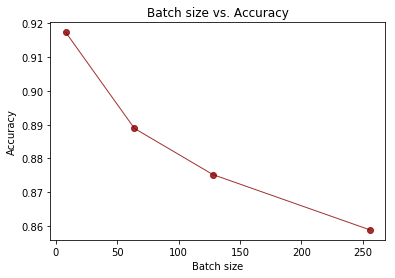In :
plt.plot(batch_size, vec_runs, linestyle='-', marker='o',
color='darkblue', linewidth=1, alpha=0.8)
plt.title("Batch size vs. execution time")
plt.ylabel('Execution time')
plt.xlabel('Batch size')
plt.show()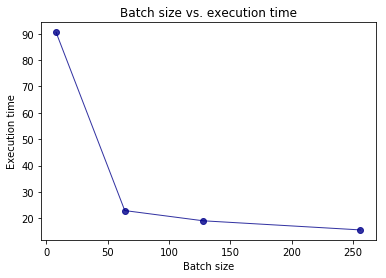Question: Similarly, vary parameter learning_rate through values 0.001, 0.005, 0.01, 0.02 and 0.05. Report and plot changes in the execution time and accuracy.

In :
# Define paramaters for the model
learning_rate = [0.001, 0.005, 0.01, 0.02, 0.05]
batch_size = 128
n_epochs = 30

In :
# Define empy arrays
vec_acc = []
vec_runs = []

# Loop trough values
for i in learning_rate:
acc, vec_run = run_training(i, batch_size, n_epochs, mnist)
vec_acc.append(acc)
vec_runs.append(vec_run)

Average loss epoch 0: 2.11109958606
Average loss epoch 1: 1.77337754301
Average loss epoch 2: 1.53053915195
Average loss epoch 3: 1.35199989556
Average loss epoch 4: 1.22085755911
Average loss epoch 5: 1.11478903535
Average loss epoch 6: 1.0406617443
Average loss epoch 7: 0.969922105332
Average loss epoch 8: 0.923886402781
Average loss epoch 9: 0.876568094556
Average loss epoch 10: 0.838552493454
Average loss epoch 11: 0.808753189646
Average loss epoch 12: 0.781183851766
Average loss epoch 13: 0.75540338474
Average loss epoch 14: 0.731005144008
Average loss epoch 15: 0.716514971151
Average loss epoch 16: 0.696597043451
Average loss epoch 17: 0.68123596337
Average loss epoch 18: 0.668232021771
Average loss epoch 19: 0.65361144187
Average loss epoch 20: 0.640719851831
Average loss epoch 21: 0.629777183066
Average loss epoch 22: 0.621821101243
Average loss epoch 23: 0.610210510956
Average loss epoch 24: 0.604856459828
Average loss epoch 25: 0.593234784467
Average loss epoch 26: 0.585182762924
Average loss epoch 27: 0.578185048692
Average loss epoch 28: 0.572447779782
Average loss epoch 29: 0.568073229078
Total time: 19.3464701176 seconds
Optimization Finished!
Accuracy 0.8759
Average loss epoch 0: 1.60237047
Average loss epoch 1: 0.988554317912
Average loss epoch 2: 0.784485932136
Average loss epoch 3: 0.682062992822
Average loss epoch 4: 0.621451242542
Average loss epoch 5: 0.578596718661
Average loss epoch 6: 0.54805848065
Average loss epoch 7: 0.526970529473
Average loss epoch 8: 0.506586670042
Average loss epoch 9: 0.48837583229
Average loss epoch 10: 0.47534381732
Average loss epoch 11: 0.468224724967
Average loss epoch 12: 0.453081037823
Average loss epoch 13: 0.444587663545
Average loss epoch 14: 0.446304094819
Average loss epoch 15: 0.427866992278
Average loss epoch 16: 0.427545563741
Average loss epoch 17: 0.42082455011
Average loss epoch 18: 0.416277818469
Average loss epoch 19: 0.412528251921
Average loss epoch 20: 0.401861042687
Average loss epoch 21: 0.404592033554
Average loss epoch 22: 0.399888946356
Average loss epoch 23: 0.393881620366
Average loss epoch 24: 0.390271394438
Average loss epoch 25: 0.390431463649
Average loss epoch 26: 0.385676200018
Average loss epoch 27: 0.381063631747
Average loss epoch 28: 0.381492746981
Average loss epoch 29: 0.378873121523
Total time: 19.7165439129 seconds
Optimization Finished!
Accuracy 0.9031
Average loss epoch 0: 1.28814772626
Average loss epoch 1: 0.730271414443
Average loss epoch 2: 0.60139799521
Average loss epoch 3: 0.536576883498
Average loss epoch 4: 0.49544279649
Average loss epoch 5: 0.473410919274
Average loss epoch 6: 0.449527029391
Average loss epoch 7: 0.437518872095
Average loss epoch 8: 0.423800003556
Average loss epoch 9: 0.410486518017
Average loss epoch 10: 0.405878430341
Average loss epoch 11: 0.393130988056
Average loss epoch 12: 0.395142771564
Average loss epoch 13: 0.380786481194
Average loss epoch 14: 0.381495635166
Average loss epoch 15: 0.373998288786
Average loss epoch 16: 0.369603976955
Average loss epoch 17: 0.36631702696
Average loss epoch 18: 0.363173119637
Average loss epoch 19: 0.358509944556
Average loss epoch 20: 0.358282115007
Average loss epoch 21: 0.351367998755
Average loss epoch 22: 0.354642130973
Average loss epoch 23: 0.346754380699
Average loss epoch 24: 0.348411404586
Average loss epoch 25: 0.34292802753
Average loss epoch 26: 0.341879764012
Average loss epoch 27: 0.342919097983
Average loss epoch 28: 0.336975129786
Average loss epoch 29: 0.335628116221
Total time: 19.3836591244 seconds
Optimization Finished!
Accuracy 0.9119
Average loss epoch 0: 1.01190109881
Average loss epoch 1: 0.571849320249
Average loss epoch 2: 0.485362586372
Average loss epoch 3: 0.442027113793
Average loss epoch 4: 0.418022875602
Average loss epoch 5: 0.401588195201
Average loss epoch 6: 0.388525825454
Average loss epoch 7: 0.377557408316
Average loss epoch 8: 0.365641438114
Average loss epoch 9: 0.36273906472
Average loss epoch 10: 0.356824811939
Average loss epoch 11: 0.348255512493
Average loss epoch 12: 0.347929626946
Average loss epoch 13: 0.338911627139
Average loss epoch 14: 0.338419038724
Average loss epoch 15: 0.337896022138
Average loss epoch 16: 0.329417232763
Average loss epoch 17: 0.332015404473
Average loss epoch 18: 0.322503993422
Average loss epoch 19: 0.327079724981
Average loss epoch 20: 0.316548404125
Average loss epoch 21: 0.32072601681
Average loss epoch 22: 0.320085886579
Average loss epoch 23: 0.319221651603
Average loss epoch 24: 0.309630358865
Average loss epoch 25: 0.316589305277
Average loss epoch 26: 0.31000279168
Average loss epoch 27: 0.311055979737
Average loss epoch 28: 0.307720559947
Average loss epoch 29: 0.303203034443
Total time: 19.3962299824 seconds
Optimization Finished!
Accuracy 0.9173
Average loss epoch 0: 0.733774306196
Average loss epoch 1: 0.442703373018
Average loss epoch 2: 0.38963984151
Average loss epoch 3: 0.37024562561
Average loss epoch 4: 0.350935442499
Average loss epoch 5: 0.339880050167
Average loss epoch 6: 0.335412788398
Average loss epoch 7: 0.326946332842
Average loss epoch 8: 0.323182789752
Average loss epoch 9: 0.317306325361
Average loss epoch 10: 0.309252664134
Average loss epoch 11: 0.309628963505
Average loss epoch 12: 0.306745398017
Average loss epoch 13: 0.302589806088
Average loss epoch 14: 0.301313768699
Average loss epoch 15: 0.297872307626
Average loss epoch 16: 0.295844274402
Average loss epoch 17: 0.294897022781
Average loss epoch 18: 0.292136274226
Average loss epoch 19: 0.2958587142
Average loss epoch 20: 0.286031504368
Average loss epoch 21: 0.292262298904
Average loss epoch 22: 0.286257720285
Average loss epoch 23: 0.287947157949
Average loss epoch 24: 0.28481074598
Average loss epoch 25: 0.284153520279
Average loss epoch 26: 0.284370262827
Average loss epoch 27: 0.279458599699
Average loss epoch 28: 0.279810138967
Average loss epoch 29: 0.283910456897
Total time: 19.5407838821 seconds
Optimization Finished!
Accuracy 0.9195

In :
print("Learning rate:", learning_rate)
print("Accuracies:", vec_acc)
print("Execution time:", vec_runs)

('Learning rate:', [0.001, 0.005, 0.01, 0.02, 0.05])
('Accuracies:', [0.87590000000000001, 0.90310000000000001, 0.91190000000000004, 0.9173, 0.91949999999999998])
('Execution time:', [19.346468925476074, 19.716542959213257, 19.383656978607178, 19.396228790283203, 19.540778875350952])

In :
plt.plot(learning_rate, vec_acc, linestyle='-', marker='o',
color='darkred', linewidth=1, alpha=0.8)
plt.title("Learning rate vs. Accuracy")
plt.ylabel('Accuracy')
plt.xlabel('Learning rate')
plt.show()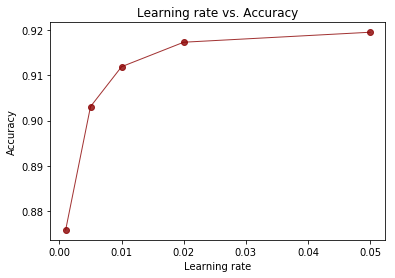In :
plt.plot(learning_rate, vec_runs, linestyle='-', marker='o',
color='darkblue', linewidth=1, alpha=0.8)
plt.title("Learning rate vs. execution time")
plt.ylabel('Execution time')
plt.xlabel('Learning rate')
plt.ylim([0, 45])

plt.show()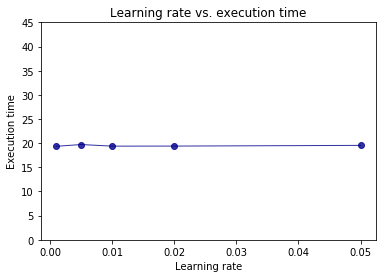# Problem 3 (35%)¶

Fetch Iris Dataset from https://archive.ics.uci.edu/ml/datasets/Iris and make attached Python script, softmax_irises.py work. You might have to upgrade the script to TF 1.x API. Generate TensorBoard graph of the process and use scalar summary to presenting variation of the loss function during the training process. Report the results of the evaluation process.

#### Get the data¶

In order to get the data we can use wget and download the iris data from the ics link above with the following command:

wget https://archive.ics.uci.edu/ml/machine-learning-databases/iris/iris.data


Next I'm upgrading the softmax_irises.py code in the following lines:

In :
# Softmax example in TF using the classical Iris dataset
import tensorflow as tf
import os


#### Set Variables¶

In :
# this time weights form a matrix, not a column vector,
# one "weight vector" per class.
W = tf.Variable(tf.zeros([4, 3]), name="weights")

# so do the biases, one per class.
b = tf.Variable(tf.zeros(, name="bias"))


#### Functions¶

In :
def combine_inputs(X):
return tf.matmul(X, W) + b

def inference(X):
return tf.nn.softmax(combine_inputs(X))

def loss(X, Y):
loss=tf.reduce_mean(tf.nn.sparse_softmax_cross_entropy_with_logits(logits=combine_inputs(X),
labels=Y))
tf.summary.scalar('loss', loss)
return loss

filename_queue = tf.train.string_input_producer(["/home/tim/hw11/data/" + "/" + file_name])

# decode_csv will convert a Tensor from type string (the text line) in
# a tuple of tensor columns with the specified defaults, which also
# sets the data type for each column
decoded = tf.decode_csv(value, record_defaults=record_defaults)

# batch actually reads the file and loads "batch_size" rows in a single tensor
return tf.train.shuffle_batch(decoded,
batch_size=batch_size,
capacity=batch_size * 50,
min_after_dequeue=batch_size)

def inputs():
sepal_length, sepal_width, petal_length, petal_width, label =\
read_csv(100, "iris.data", [[0.0], [0.0], [0.0], [0.0], [""]])

# convert class names to a 0 based class index.
label_number = tf.to_int32(tf.argmax(tf.to_int32(tf.stack([
tf.equal(label, ["Iris-setosa"]),
tf.equal(label, ["Iris-versicolor"]),
tf.equal(label, ["Iris-virginica"])
])), 0))

# Pack all the features that we care about in a single matrix;
# We then transpose to have a matrix with one example per row and one feature per column.
features = tf.transpose(tf.stack([sepal_length, sepal_width,
petal_length, petal_width]))

return features, label_number

def train(total_loss):
learning_rate = 0.01

def evaluate(sess, X, Y):
predicted = tf.cast(tf.argmax(inference(X), 1), tf.int32)
print sess.run(tf.reduce_mean(tf.cast(tf.equal(predicted, Y), tf.float32)))


#### Train model¶

In :
# Launch the graph in a session, setup boilerplate
with tf.Session() as sess:
tf.global_variables_initializer().run()

X, Y = inputs()
total_loss = loss(X, Y)
train_op = train(total_loss)

coord = tf.train.Coordinator()

# actual training loop
training_steps = 1000
for step in range(training_steps):
sess.run([train_op])
# for debugging and learning purposes, see how the loss gets decremented thru training steps
if step % 10 == 0:

print "loss: ", sess.run([total_loss])

writer = tf.summary.FileWriter('/home/tim/hw11/p3_iris', sess.graph)
evaluate(sess, X, Y)
writer.flush()
writer.close()
coord.request_stop()
sess.close()

loss:  [1.0933079]
loss:  [1.0129499]
loss:  [0.96216154]
loss:  [0.90950936]
loss:  [0.86003435]
loss:  [0.84646928]
loss:  [0.81177109]
loss:  [0.76787591]
loss:  [0.78017074]
loss:  [0.68854356]
loss:  [0.72085834]
loss:  [0.72047102]
loss:  [0.66443521]
loss:  [0.66118151]
loss:  [0.65529788]
loss:  [0.65343076]
loss:  [0.68200105]
loss:  [0.60359037]
loss:  [0.60067827]
loss:  [0.61667144]
loss:  [0.54109991]
loss:  [0.61589342]
loss:  [0.56667554]
loss:  [0.5322088]
loss:  [0.58395886]
loss:  [0.52062112]
loss:  [0.52611327]
loss:  [0.54074407]
loss:  [0.50503832]
loss:  [0.5667752]
loss:  [0.5416916]
loss:  [0.45924258]
loss:  [0.52459204]
loss:  [0.48514757]
loss:  [0.53373694]
loss:  [0.46871611]
loss:  [0.47602504]
loss:  [0.53625083]
loss:  [0.46304154]
loss:  [0.48620269]
loss:  [0.49945885]
loss:  [0.45058793]
loss:  [0.49342698]
loss:  [0.43937066]
loss:  [0.49195588]
loss:  [0.47834265]
loss:  [0.43595183]
loss:  [0.42401549]
loss:  [0.47928536]
loss:  [0.42596015]
loss:  [0.48529303]
loss:  [0.42815438]
loss:  [0.43521243]
loss:  [0.45617977]
loss:  [0.43579113]
loss:  [0.44305092]
loss:  [0.45701736]
loss:  [0.44056726]
loss:  [0.45121452]
loss:  [0.39159188]
loss:  [0.44377488]
loss:  [0.42469341]
loss:  [0.37185028]
loss:  [0.4351548]
loss:  [0.3972708]
loss:  [0.41297051]
loss:  [0.46545514]
loss:  [0.37948066]
loss:  [0.42458642]
loss:  [0.38890326]
loss:  [0.38554573]
loss:  [0.41644919]
loss:  [0.39299759]
loss:  [0.41099191]
loss:  [0.40212375]
loss:  [0.39310658]
loss:  [0.42595708]
loss:  [0.40580696]
loss:  [0.37521157]
loss:  [0.38816079]
loss:  [0.36049789]
loss:  [0.39589867]
loss:  [0.38728839]
loss:  [0.36019307]
loss:  [0.41735673]
loss:  [0.35798699]
loss:  [0.36043414]
loss:  [0.40018418]
loss:  [0.35495013]
loss:  [0.35962921]
loss:  [0.36168388]
loss:  [0.3609868]
loss:  [0.39332759]
loss:  [0.34980679]
loss:  [0.37539724]
loss:  [0.35697278]
loss:  [0.35106277]
loss:  [0.37394392]
loss:  [0.33326298]
loss:  [0.37630051]
1.0


Above we see the output from the adopted code. Next we can also have a look at the tensorgraph.

### Plot Tensorgraph¶

tensorboard --logdir 'p3_iris'

In :
fig = Image(filename=('/home/tim/hw11/img/p3_1.png'))
fig

Out: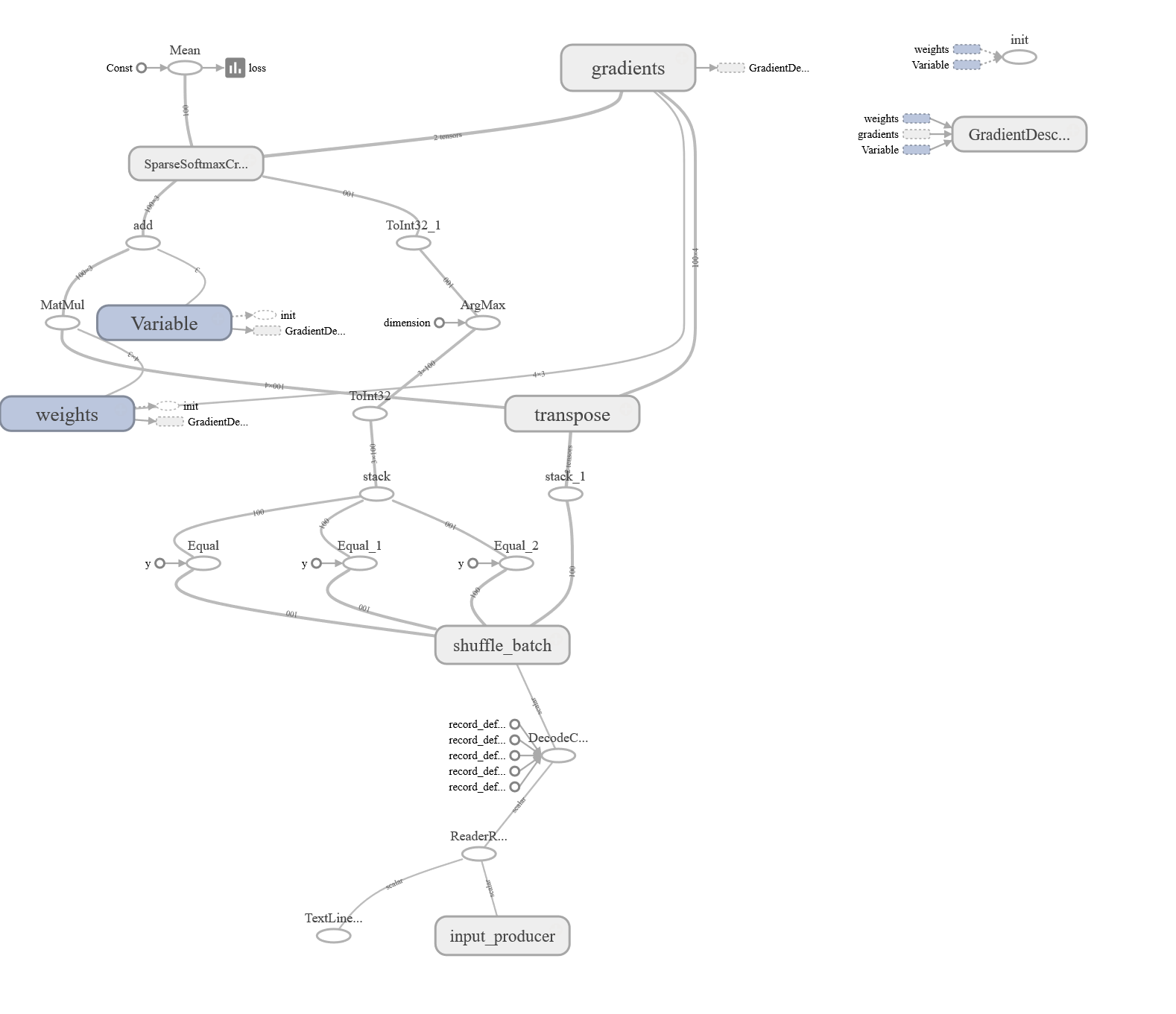# Problem 4 (25%)¶

Analyze all relevant and non-obvious individual steps in the script, softwmax_irises.py by examining their inputs and outputs. When convenient, use existing Iris Dataset. When convenient, you are welcome to provide your own inputs. Please examine and describe actions of functions and operations within those functions. Please describe the effect of every function or command by providing an illustrative input and output set of values and well as a brief narrative.

### Iris data¶

Taking the iris dataset we can have a look at the transformations happening. After reading the data we have four 100 x 1 tensors (features) and an additional 100 x 1 tensor (label). The initial dependent variable tensor contains text descriptions for the 3 species iris-setosa, iris-versicolar and iris-virginica. Those are converted into a 0 1 2 tensor. This looks like this:

#### X tensor (3 rows)¶

[5.4000001 3.70000005 1.5        0.2]
[4.5999999 3.20000005 1.39999998 0.2]
[5.4000001 3.         4.5        1.5]


#### labels¶

['Iris-setosa' 'Iris-setosa' 'Iris-versicolor' 'Iris-setosa' 'Iris-setosa'
'Iris-setosa' 'Iris-setosa' 'Iris-versicolor' 'Iris-setosa' 'Iris-setosa'
'Iris-versicolor' 'Iris-versicolor' 'Iris-setosa' 'Iris-versicolor'
'Iris-versicolor' 'Iris-versicolor' 'Iris-versicolor' 'Iris-virginica'
'Iris-setosa' 'Iris-setosa' 'Iris-versicolor' 'Iris-setosa'
'Iris-virginica' 'Iris-virginica' 'Iris-setosa' 'Iris-setosa'
'Iris-versicolor' 'Iris-virginica' 'Iris-versicolor' 'Iris-versicolor'
'Iris-setosa' 'Iris-setosa' 'Iris-versicolor' 'Iris-setosa'
'Iris-virginica' 'Iris-versicolor' 'Iris-versicolor' 'Iris-versicolor'
'Iris-virginica' 'Iris-virginica' 'Iris-versicolor' 'Iris-virginica'
'Iris-virginica' 'Iris-setosa' 'Iris-setosa' 'Iris-setosa'
'Iris-virginica' 'Iris-setosa' 'Iris-versicolor' 'Iris-setosa'
'Iris-versicolor' 'Iris-setosa' 'Iris-virginica' 'Iris-setosa'
'Iris-virginica' 'Iris-virginica' 'Iris-versicolor' 'Iris-setosa'
'Iris-virginica' 'Iris-versicolor' 'Iris-setosa' 'Iris-virginica'
'Iris-virginica' 'Iris-virginica' 'Iris-setosa' 'Iris-virginica'
'Iris-virginica' 'Iris-setosa' 'Iris-setosa' 'Iris-setosa'
'Iris-versicolor' 'Iris-setosa' 'Iris-setosa' 'Iris-setosa'
'Iris-versicolor' 'Iris-virginica' 'Iris-virginica' 'Iris-virginica'
'Iris-virginica' 'Iris-versicolor' 'Iris-versicolor' 'Iris-setosa'
'Iris-virginica' 'Iris-virginica' 'Iris-versicolor' 'Iris-setosa'
'Iris-virginica' 'Iris-virginica' 'Iris-versicolor' 'Iris-setosa'
'Iris-versicolor' 'Iris-setosa' 'Iris-setosa' 'Iris-setosa' 'Iris-setosa'
'Iris-setosa' 'Iris-setosa' 'Iris-setosa' 'Iris-versicolor' 'Iris-setosa']


#### label tensors¶

[0 0 1 0 0 0 0 1 0 0 1 1 0 1 1 1 1 2 0 0 1 0 2 2 0 0 1 2 1 1 0 0 1 0 2 1 1
1 2 2 1 2 2 0 0 0 2 0 1 0 1 0 2 0 2 2 1 0 2 1 0 2 2 2 0 2 2 0 0 0 1 0 0 0
1 2 2 2 2 1 1 0 2 2 1 0 2 2 1 0 1 0 0 0 0 0 0 0 1 0]


### Functions¶

Next we can have a look at the individual functions.

combine_inputs(), line 13


The combine_inputs() function brings together the inputs, weights and bias to calculate the Y(output) vector for the given input features. That means the functions takes X as an argument and returns X*W + b. Where W is the weights and b the bias as a constant.

inference(), line 17


This inference function calls up the softmax implementation of tensorflow. That means the function calculates the probability the input vector calculated by the above combine_inputs() function.

read_csv(), line 25


The above read_csv function reads in the csv document we want to load. In order to do that it takes 3 arguments (size of the batch, name of the file and the default records to be used when the text is a string.

decode_csv() line 34


The above decode_csv function takes the input file and transfroms the string type into a tensor with columns. When we use the function in our case of the iris set a tensor with 5 columns is resulting as an output. The 4 first columns are encoded as float32 and the label is encoded as a string.

train.shuffle_batch(), line 37


The train.shuffle_batch function randomly shuffles the tensor and trought that creates batches. It returns a list of dictionary of tensors with the type as tensors.

inputs(), line 43


The purpose of the input function is to prepare the 4 features and the 1 label.

label_number = tf.to_int32(…), line 49


The label_number function converts the string class names to integer labels. This can also be seen when looking at the full line:

label_number = tf.to_int32(tf.argmax(tf.to_int32(tf.stack([tf.equal(label, ["Iris-setosa"]),tf.equal(label, ["Iris-versicolor"]),tf.equal(label, ["Iris-virginica"])])), 0))


We can see that the function identifies the potential classes (values of label) and ultimately converts them to values of Integer type (0-based).

features = tf.transpose(..), line 57


The above function take all 4 input features from the csv file and puts them into a single matrix. Next it transposes the matrix with one example per row and one feature per column.

evaluate(), line 67


The above function predicts values based on the model and then compares the predicted labels against the actual labels.

predicted = tf.cast(tf.arg_max(inference(X), 1).., line 69


This line of Evaluate function calls inference with the input vector as input. The inference function inturn calculates the probability the input vector belongs to each class. The arg_max is used to predict the label based on the max of the output vector.

tf.reduce_mean(tf.cast(tf.equal(predicted,Y),.,line 71


The above function calculates the mean of the difference between predicted and the actual class for each input vector. In other words we're measuring the accuracy of the model.

threads = tf.train.start_queue_runners(sess=sess, coord=coord).., line 85


The above function start the queue runners collected in the graph, and it returns the list of all threads. These threads use a coordinator to stop together, i.e., the coordinator helps the multiple threads to stop together.